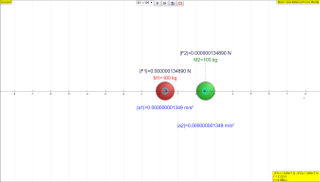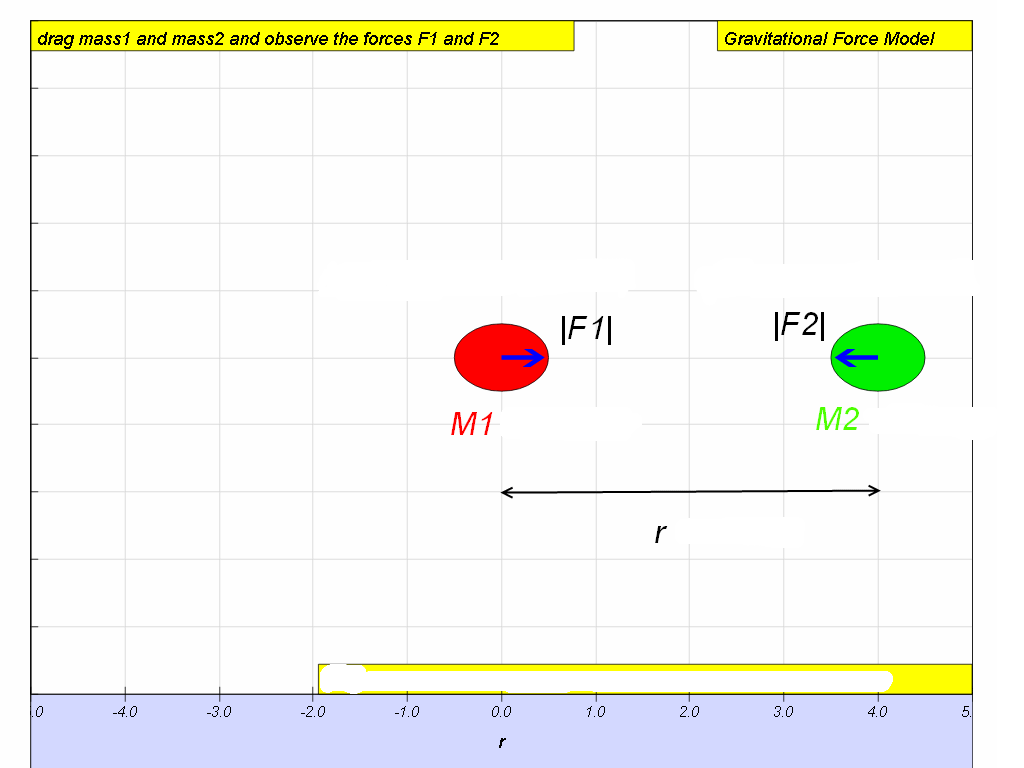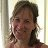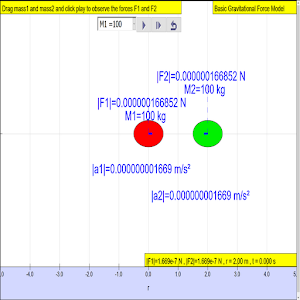gravity01

7.1 Newton’s Law of Gravitation

Gravitation is a natural phenomenon by which physical bodies attract each other due to their masses. This force occurs whenever masses are present and the two bodies need not to be in contact with each other. It is however the weakest of the fundamental forces of nature.

In 1687, Sir Isaac Newton concluded that this non-contact gravitational force must be as responsible for the falling of the apple from a tree as it is the cause for the rotation of the Moon about the Earth. Hence he published the Newton’s law of gravitation which states that:

“The mutual force of attraction between any two point masses is directly proportional to the product of their masses and inversely proportional to the square of the separation between their centres.”This means that if there are two point masses M and m and they are separated by distance r, the magnitude of the gravitational force attracting them to each other is$|F|=\frac{G{M}_{1}{M}_{2}}{{r}^{2}}$   where G, the constant of universal gravitation, is $6.\text{67x1}{0}^{-11}\frac{N{m}^{2}}{k{g}^{2}}$ (will be given in data & formulae list during tests and examinations).

Note:
1.    r is taken to be the centre to centre distance (i.e. centre of particle to centre of particle). Do not take r to be the radius of orbit!
2.    This formula is an example of the inverse square law.

7.1.1 Inquiry:

What can you conclude about the two forces in the simulation below?

Answer: Based on the evidences from the simulation triangulated with real life examples, the mutual force of attraction between any two point masses is directly proportional to the product of their masses and inversely proportional to the square of the separation between their centres.

Translations

Code Language Translator RunSoftware Requirements

SoftwareRequirements

 Android iOS Windows MacOS with best with Chrome Chrome Chrome Chrome support full-screen? Yes. Chrome/Opera No. Firefox/ Samsung Internet Not yet Yes Yes cannot work on some mobile browser that don't understand JavaScript such as..... cannot work on Internet Explorer 9 and below

CreditsThis email address is being protected from spambots. You need JavaScript enabled to view it.; Anne Cox; Wolfgang Christian; Francisco Esquembre

end faq

http://iwant2study.org/lookangejss/02_newtonianmechanics_7gravity/ejss_model_gravity01_0/gravity01_0_Simulation.xhtml

Appshttps://play.google.com/store/apps/details?id=com.ionicframework.gravity010app251517&hl=en

Sample Learning Goals

1. recall and use Newton's law of gravitation in the form $$|F| = \frac{M_{1}M_{2}}{r^{2}}$$

Research 6.5 Gravitational field: Map of key concepts and ideas covered at upper secondary level Newton’s law of universal gravitation Key inquiry question:

How can we express the gravitational interaction between objects in the Universe?

1. Gravitational field

• A gravitational field is a region in which a mass experiences a force due to gravitational attraction.

• Gravitational field strength, g of a field is defined as the gravitational force per unit mass.

2. Field near to the surface of the Earth

• The acceleration of free fall for a body near to the Earth is constant and is approximately 10 m/s2 .

Students’ prior knowledge of Dynamics and Gravitational field

Primary level:

Students learn about mass (a measure of the amount of matter in a body) and volume (the amount of space that a body occupies) and the use of appropriate apparatus to measure these quantities (e.g. use of a lever balance, an electronic balance, a measuring cylinder, a syringe, and a measuring jug). However, the concept of density is not introduced although students do simple experiments to investigate the ability of objects of different materials (plastics, wood, rubber and metals) to float/sink in water.

Students recognize that objects have weight because of the gravitational force between them and the Earth and that weight is different at different places, and can be measured using a spring balance or a weighing scale.

Lower secondary level:

Students learn that:

• the density of a substance is the mass of the substance per unit volume, and can be used to predict whether objects sink or float.

• gravity exists between any two objects (e.g. ball and Earth). The weight of an object depends on the force of gravity pulling on that object. Students’ common misconceptions and learning difficulties in Dynamics and Gravitational field

Newton’s first law:

Students often think that:

• a force is required to maintain an object in its motion;

• if there is no motion, there is no force acting;

• constant speed results from a constant force;

• friction (instead of inertia) causes objects to resist a change in its state of rest or motion.

Newton’s second law:

Students often think that:

• a larger velocity means a larger resultant force;

• acceleration implies increasing force;

• greater mass implies greater force;

• a force cannot move an object unless it is greater than the object’s weight;

• heavier objects fall faster than light objects.

Newton’s third law:

Students often think that force is a single physical quantity associated with a single object rather than as an interaction between two objects which must therefore exist as an action reaction pair. They have difficulty in understanding that:

• two objects of greatly differing masses (e.g. Earth and us) exert forces of equal magnitude on each other;

• the normal force on an object and the weight of the object do not always have equal magnitudes;

• gravity acts on an object all the time (not just when it is falling).

Video

7.1 Gravity Force Model (Basic) JavaScript by lookang lawrence wee

Version:

1. http://weelookang.blogspot.sg/2016/03/a-comparison-of-phet-and-osp-gravity-lab.html refreshed version
2. http://weelookang.blogspot.sg/2015/04/ejss-gravity-022.html?q=gravity earilest post by lookang
3. http://www.opensourcephysics.org/items/detail.cfm?ID=9683 based on Coulomb's Law and Electric Field Package by Anne Cox, Wolfgang Christian, and Francisco Esquembre

end faqRating 5.00 (5 Votes)